# Team:SMS Shenzhen/Model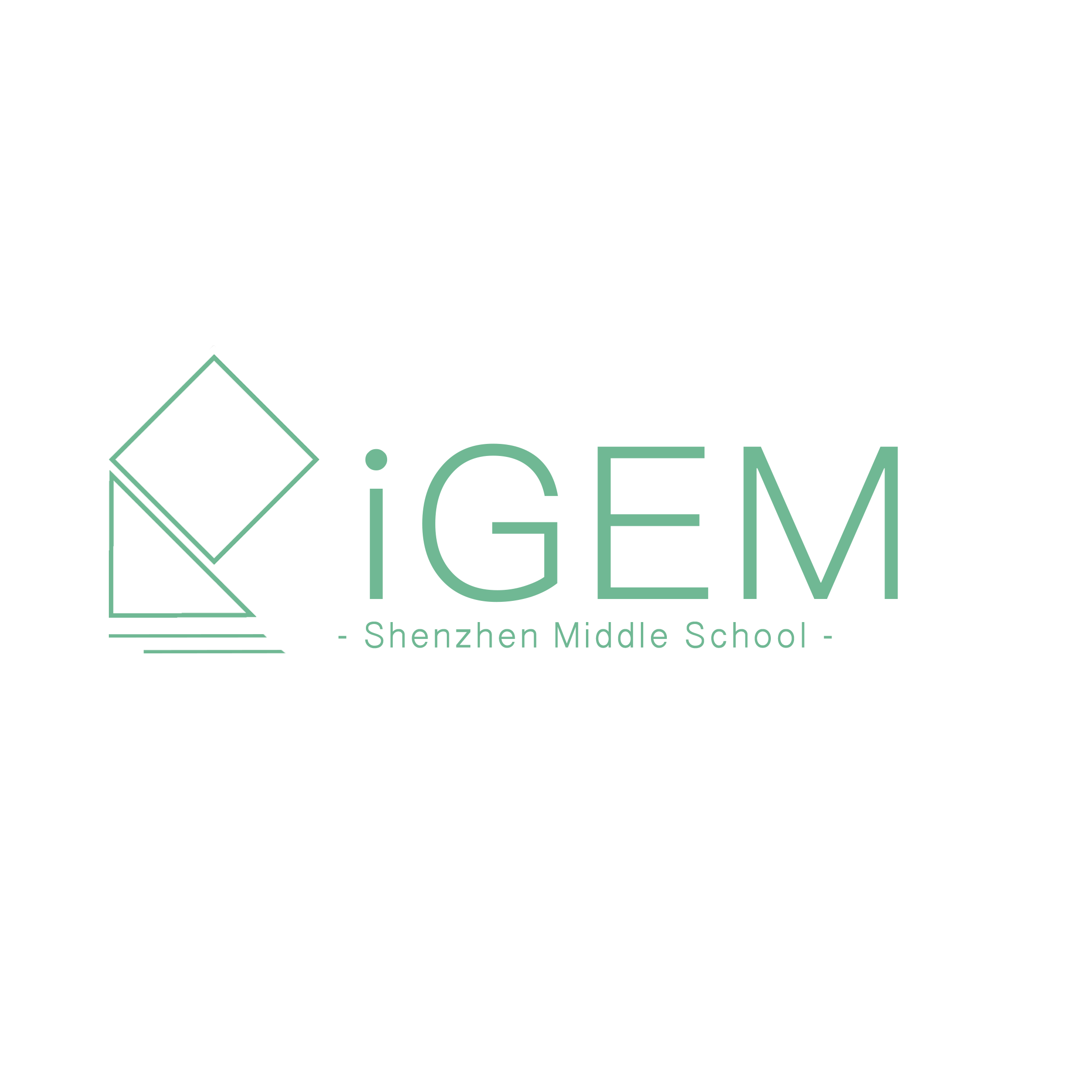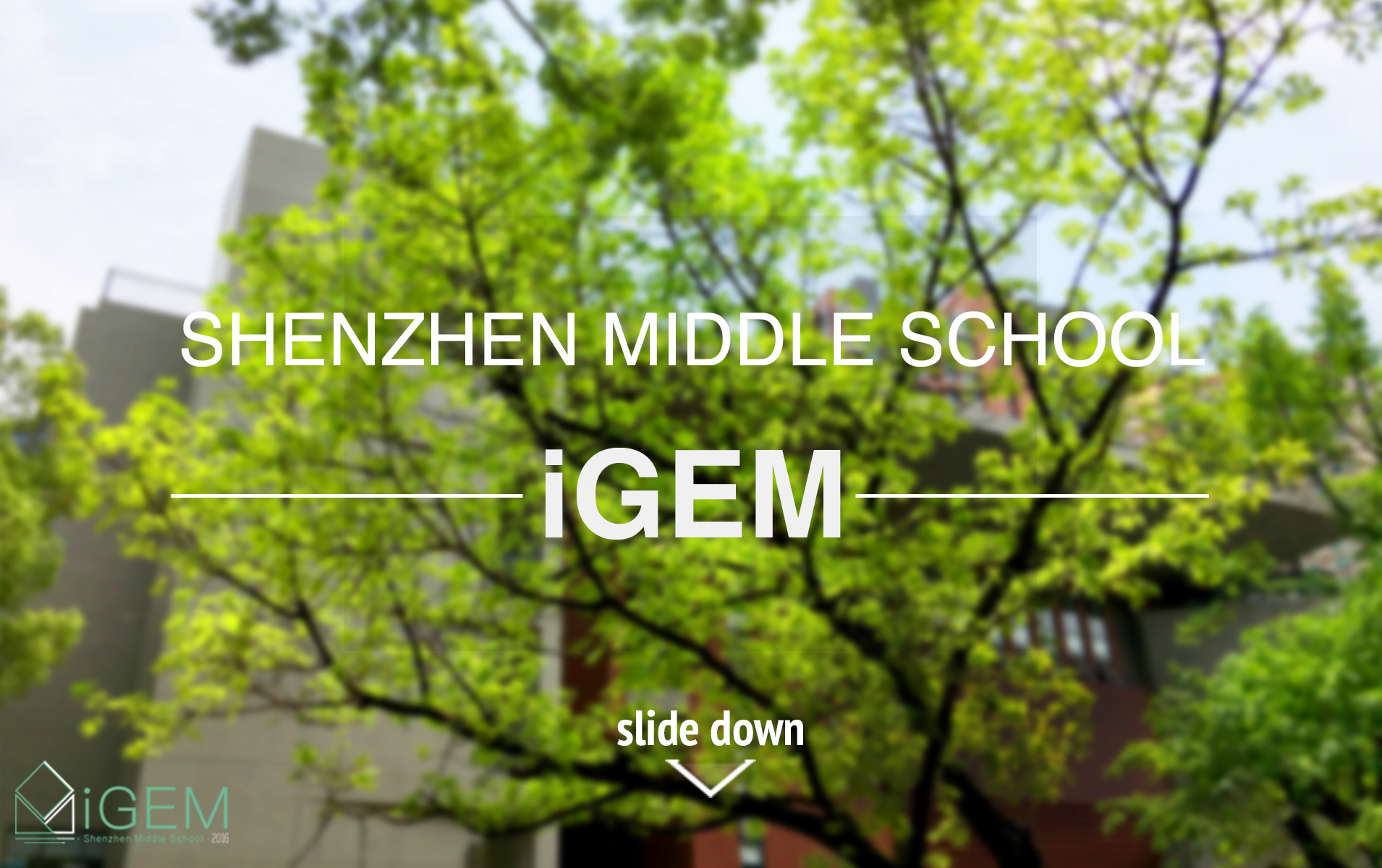# SMS: Mathematical Modeling

## 1.Translation System

### Consider a deterministic model for the central theorem.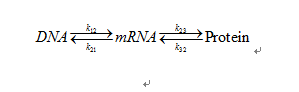### We assume the reactions are homogenous, not compartmented, and are all 1st order reactions. Therefore, we may obtain the following model equations. In the model equation, we denote DNA as A, mRNA as B, and protein as C.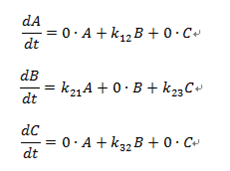### The parameters of the model equations: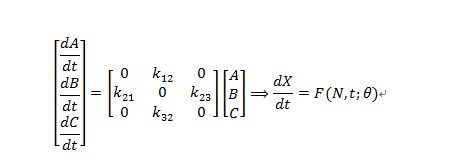### These equations can be written in matric form:## 1.iPTG Induction Model

### Culture medium volume can be calculated using the difference between V and V_cel.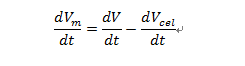### iPTG evolution can be written as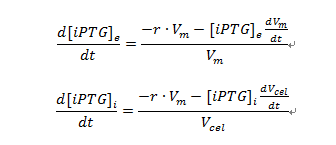### These two equations describe the variation with time of extracellular and intracellular IPTG concentration (subindex “e” denotes extracellular and sub-index “i” denotes intracellular) as function of the net transport rate (r) and dilution (first and second term on the right side). These two equations can both be derived using mass balances as shown below: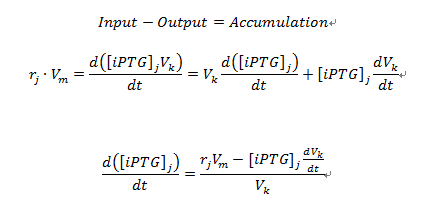### In which